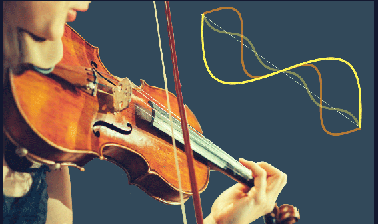Ir al contenido principal

# Differential Equations: Fourier Series and Partial Differential Equations

Learn to use Fourier series to solve differential equations with periodic input signals and to solve boundary value problems involving the heat equation and wave equation.

...